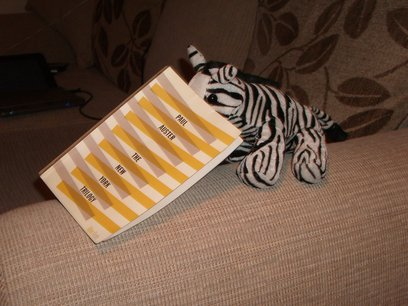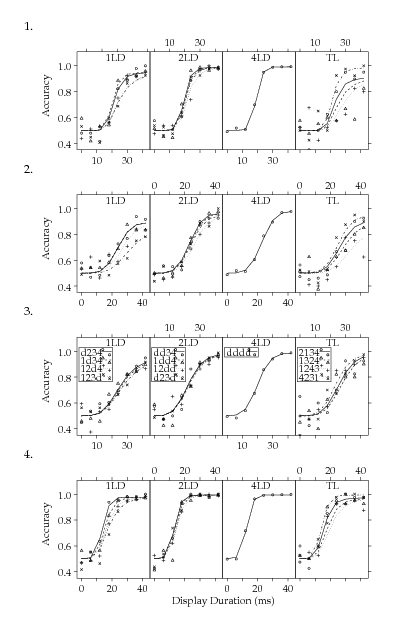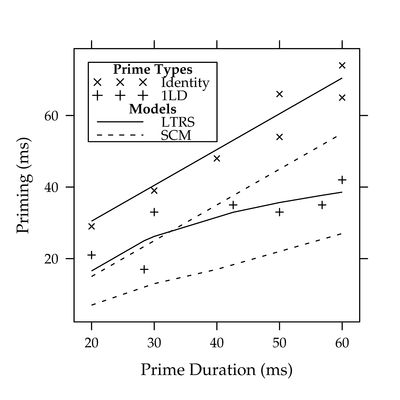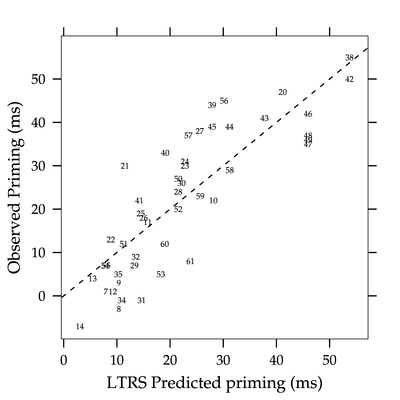# Letters in Time and Retinotopic Space (LTRS)

Adelman, J. S. (in press). Letters in Time and Retinotopic Space. Psychological Review.

This code uses the known exact mathematical properties of the model and numerical integration to produce exact proportion known and average priming predictions. A true simulation would involve simpler code, but would require a massive number of runs to average out the trial-to-trial variability involved in a stochastic model.Graph Data Predictions Match scores R Code for fits/guessing READMEText tables:

Fitted data:

Practice data:

LTRS: <- use models R code (uses shared library above)
(R part implements guessing)Text table

Overlap model
(original fits, 31 pars:
new pars for each expt)
Spatial Coding,
footnote
R code (uses shared library above)
(R part implements guessing)Text tableText table